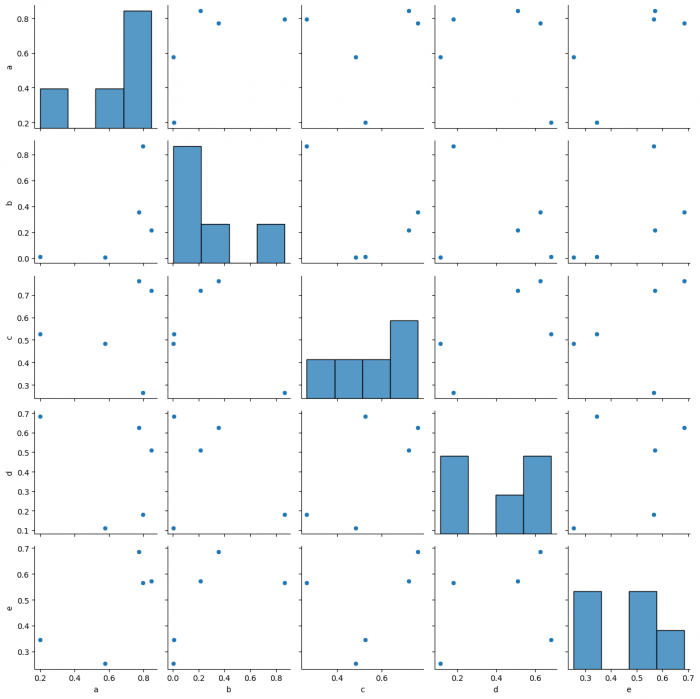# How to save a plot in Seaborn with Python (Matplotlib)?

To save a plot in Seaborn, we can use the savefig() method.

## Steps

• Set the figure size and adjust the padding between and around the subplots.
• Make a two-dimensional, size-mutable, potentially heterogeneous tabular data.
• Plot pairwise relationships in a dataset.
• Save the plot into a file using savefig() method.
• To display the figure, use show() method.

## Example

import seaborn as sns
import pandas as pd
import numpy as np
import matplotlib.pyplot as plt

plt.rcParams["figure.figsize"] = [7.50, 3.50]
plt.rcParams["figure.autolayout"] = True

df = pd.DataFrame(np.random.random((5, 5)), columns=["a", "b", "c", "d", "e"])
sns_pp = sns.pairplot(df)
sns_pp.savefig("sns-heatmap.png")

## Output

When we execute the code, it will create the following plot and save it as "sns-heatmap.png" in the project folder.